# `sklearn.tree`.DecisionTreeClassifier¶

class `sklearn.tree.``DecisionTreeClassifier`(criterion='gini', splitter='best', max_depth=None, min_samples_split=2, min_samples_leaf=1, min_weight_fraction_leaf=0.0, max_features=None, random_state=None, max_leaf_nodes=None, min_impurity_split=1e-07, class_weight=None, presort=False)[source]

A decision tree classifier.

Read more in the User Guide.

Parameters: criterion : string, optional (default=”gini”) The function to measure the quality of a split. Supported criteria are “gini” for the Gini impurity and “entropy” for the information gain. splitter : string, optional (default=”best”) The strategy used to choose the split at each node. Supported strategies are “best” to choose the best split and “random” to choose the best random split. max_features : int, float, string or None, optional (default=None) The number of features to consider when looking for the best split: If int, then consider max_features features at each split. If float, then max_features is a percentage and int(max_features * n_features) features are considered at each split. If “auto”, then max_features=sqrt(n_features). If “sqrt”, then max_features=sqrt(n_features). If “log2”, then max_features=log2(n_features). If None, then max_features=n_features. Note: the search for a split does not stop until at least one valid partition of the node samples is found, even if it requires to effectively inspect more than `max_features` features. max_depth : int or None, optional (default=None) The maximum depth of the tree. If None, then nodes are expanded until all leaves are pure or until all leaves contain less than min_samples_split samples. min_samples_split : int, float, optional (default=2) The minimum number of samples required to split an internal node: If int, then consider min_samples_split as the minimum number. If float, then min_samples_split is a percentage and ceil(min_samples_split * n_samples) are the minimum number of samples for each split. Changed in version 0.18: Added float values for percentages. min_samples_leaf : int, float, optional (default=1) The minimum number of samples required to be at a leaf node: If int, then consider min_samples_leaf as the minimum number. If float, then min_samples_leaf is a percentage and ceil(min_samples_leaf * n_samples) are the minimum number of samples for each node. Changed in version 0.18: Added float values for percentages. min_weight_fraction_leaf : float, optional (default=0.) The minimum weighted fraction of the sum total of weights (of all the input samples) required to be at a leaf node. Samples have equal weight when sample_weight is not provided. max_leaf_nodes : int or None, optional (default=None) Grow a tree with `max_leaf_nodes` in best-first fashion. Best nodes are defined as relative reduction in impurity. If None then unlimited number of leaf nodes. class_weight : dict, list of dicts, “balanced” or None, optional (default=None) Weights associated with classes in the form `{class_label: weight}`. If not given, all classes are supposed to have weight one. For multi-output problems, a list of dicts can be provided in the same order as the columns of y. The “balanced” mode uses the values of y to automatically adjust weights inversely proportional to class frequencies in the input data as `n_samples / (n_classes * np.bincount(y))` For multi-output, the weights of each column of y will be multiplied. Note that these weights will be multiplied with sample_weight (passed through the fit method) if sample_weight is specified. random_state : int, RandomState instance or None, optional (default=None) If int, random_state is the seed used by the random number generator; If RandomState instance, random_state is the random number generator; If None, the random number generator is the RandomState instance used by np.random. min_impurity_split : float, optional (default=1e-7) Threshold for early stopping in tree growth. A node will split if its impurity is above the threshold, otherwise it is a leaf. New in version 0.18. presort : bool, optional (default=False) Whether to presort the data to speed up the finding of best splits in fitting. For the default settings of a decision tree on large datasets, setting this to true may slow down the training process. When using either a smaller dataset or a restricted depth, this may speed up the training. classes_ : array of shape = [n_classes] or a list of such arrays The classes labels (single output problem), or a list of arrays of class labels (multi-output problem). feature_importances_ : array of shape = [n_features] The feature importances. The higher, the more important the feature. The importance of a feature is computed as the (normalized) total reduction of the criterion brought by that feature. It is also known as the Gini importance [R245]. max_features_ : int, The inferred value of max_features. n_classes_ : int or list The number of classes (for single output problems), or a list containing the number of classes for each output (for multi-output problems). n_features_ : int The number of features when `fit` is performed. n_outputs_ : int The number of outputs when `fit` is performed. tree_ : Tree object The underlying Tree object.

References

 [R243] L. Breiman, J. Friedman, R. Olshen, and C. Stone, “Classification and Regression Trees”, Wadsworth, Belmont, CA, 1984.
 [R244] T. Hastie, R. Tibshirani and J. Friedman. “Elements of Statistical Learning”, Springer, 2009.
 [R245] (1, 2) L. Breiman, and A. Cutler, “Random Forests”, http://www.stat.berkeley.edu/~breiman/RandomForests/cc_home.htm

Examples

```>>> from sklearn.datasets import load_iris
>>> from sklearn.model_selection import cross_val_score
>>> from sklearn.tree import DecisionTreeClassifier
>>> clf = DecisionTreeClassifier(random_state=0)
>>> cross_val_score(clf, iris.data, iris.target, cv=10)
...
...
array([ 1.     ,  0.93...,  0.86...,  0.93...,  0.93...,
0.93...,  0.93...,  1.     ,  0.93...,  1.      ])
```

Methods

 `apply`(X[, check_input]) Returns the index of the leaf that each sample is predicted as. `decision_path`(X[, check_input]) Return the decision path in the tree `fit`(X, y[, sample_weight, check_input, ...]) Build a decision tree classifier from the training set (X, y). `fit_transform`(X[, y]) Fit to data, then transform it. `get_params`([deep]) Get parameters for this estimator. `predict`(X[, check_input]) Predict class or regression value for X. `predict_log_proba`(X) Predict class log-probabilities of the input samples X. `predict_proba`(X[, check_input]) Predict class probabilities of the input samples X. `score`(X, y[, sample_weight]) Returns the mean accuracy on the given test data and labels. `set_params`(\*\*params) Set the parameters of this estimator. `transform`(\*args, \*\*kwargs) DEPRECATED: Support to use estimators as feature selectors will be removed in version 0.19.
`__init__`(criterion='gini', splitter='best', max_depth=None, min_samples_split=2, min_samples_leaf=1, min_weight_fraction_leaf=0.0, max_features=None, random_state=None, max_leaf_nodes=None, min_impurity_split=1e-07, class_weight=None, presort=False)[source]
`apply`(X, check_input=True)[source]

Returns the index of the leaf that each sample is predicted as.

New in version 0.17.

Parameters: X : array_like or sparse matrix, shape = [n_samples, n_features] The input samples. Internally, it will be converted to `dtype=np.float32` and if a sparse matrix is provided to a sparse `csr_matrix`. check_input : boolean, (default=True) Allow to bypass several input checking. Don’t use this parameter unless you know what you do. X_leaves : array_like, shape = [n_samples,] For each datapoint x in X, return the index of the leaf x ends up in. Leaves are numbered within `[0; self.tree_.node_count)`, possibly with gaps in the numbering.
`decision_path`(X, check_input=True)[source]

Return the decision path in the tree

New in version 0.18.

Parameters: X : array_like or sparse matrix, shape = [n_samples, n_features] The input samples. Internally, it will be converted to `dtype=np.float32` and if a sparse matrix is provided to a sparse `csr_matrix`. check_input : boolean, (default=True) Allow to bypass several input checking. Don’t use this parameter unless you know what you do. indicator : sparse csr array, shape = [n_samples, n_nodes] Return a node indicator matrix where non zero elements indicates that the samples goes through the nodes.
`feature_importances_`

Return the feature importances.

The importance of a feature is computed as the (normalized) total reduction of the criterion brought by that feature. It is also known as the Gini importance.

Returns: feature_importances_ : array, shape = [n_features]
`fit`(X, y, sample_weight=None, check_input=True, X_idx_sorted=None)[source]

Build a decision tree classifier from the training set (X, y).

Parameters: X : array-like or sparse matrix, shape = [n_samples, n_features] The training input samples. Internally, it will be converted to `dtype=np.float32` and if a sparse matrix is provided to a sparse `csc_matrix`. y : array-like, shape = [n_samples] or [n_samples, n_outputs] The target values (class labels) as integers or strings. sample_weight : array-like, shape = [n_samples] or None Sample weights. If None, then samples are equally weighted. Splits that would create child nodes with net zero or negative weight are ignored while searching for a split in each node. Splits are also ignored if they would result in any single class carrying a negative weight in either child node. check_input : boolean, (default=True) Allow to bypass several input checking. Don’t use this parameter unless you know what you do. X_idx_sorted : array-like, shape = [n_samples, n_features], optional The indexes of the sorted training input samples. If many tree are grown on the same dataset, this allows the ordering to be cached between trees. If None, the data will be sorted here. Don’t use this parameter unless you know what to do. self : object Returns self.
`fit_transform`(X, y=None, **fit_params)[source]

Fit to data, then transform it.

Fits transformer to X and y with optional parameters fit_params and returns a transformed version of X.

Parameters: X : numpy array of shape [n_samples, n_features] Training set. y : numpy array of shape [n_samples] Target values. X_new : numpy array of shape [n_samples, n_features_new] Transformed array.
`get_params`(deep=True)[source]

Get parameters for this estimator.

Parameters: deep : boolean, optional If True, will return the parameters for this estimator and contained subobjects that are estimators. params : mapping of string to any Parameter names mapped to their values.
`predict`(X, check_input=True)[source]

Predict class or regression value for X.

For a classification model, the predicted class for each sample in X is returned. For a regression model, the predicted value based on X is returned.

Parameters: X : array-like or sparse matrix of shape = [n_samples, n_features] The input samples. Internally, it will be converted to `dtype=np.float32` and if a sparse matrix is provided to a sparse `csr_matrix`. check_input : boolean, (default=True) Allow to bypass several input checking. Don’t use this parameter unless you know what you do. y : array of shape = [n_samples] or [n_samples, n_outputs] The predicted classes, or the predict values.
`predict_log_proba`(X)[source]

Predict class log-probabilities of the input samples X.

Parameters: X : array-like or sparse matrix of shape = [n_samples, n_features] The input samples. Internally, it will be converted to `dtype=np.float32` and if a sparse matrix is provided to a sparse `csr_matrix`. p : array of shape = [n_samples, n_classes], or a list of n_outputs such arrays if n_outputs > 1. The class log-probabilities of the input samples. The order of the classes corresponds to that in the attribute classes_.
`predict_proba`(X, check_input=True)[source]

Predict class probabilities of the input samples X.

The predicted class probability is the fraction of samples of the same class in a leaf.

check_input
: boolean, (default=True)
Allow to bypass several input checking. Don’t use this parameter unless you know what you do.
Parameters: X : array-like or sparse matrix of shape = [n_samples, n_features] The input samples. Internally, it will be converted to `dtype=np.float32` and if a sparse matrix is provided to a sparse `csr_matrix`. p : array of shape = [n_samples, n_classes], or a list of n_outputs such arrays if n_outputs > 1. The class probabilities of the input samples. The order of the classes corresponds to that in the attribute classes_.
`score`(X, y, sample_weight=None)[source]

Returns the mean accuracy on the given test data and labels.

In multi-label classification, this is the subset accuracy which is a harsh metric since you require for each sample that each label set be correctly predicted.

Parameters: X : array-like, shape = (n_samples, n_features) Test samples. y : array-like, shape = (n_samples) or (n_samples, n_outputs) True labels for X. sample_weight : array-like, shape = [n_samples], optional Sample weights. score : float Mean accuracy of self.predict(X) wrt. y.
`set_params`(**params)[source]

Set the parameters of this estimator.

The method works on simple estimators as well as on nested objects (such as pipelines). The latter have parameters of the form `<component>__<parameter>` so that it’s possible to update each component of a nested object.

Returns: self :
`transform`(*args, **kwargs)[source]

DEPRECATED: Support to use estimators as feature selectors will be removed in version 0.19. Use SelectFromModel instead.

Reduce X to its most important features.

Uses `coef_` or `feature_importances_` to determine the most important features. For models with a `coef_` for each class, the absolute sum over the classes is used.
Parameters: X : array or scipy sparse matrix of shape [n_samples, n_features] The input samples. threshold : string, float or None, optional (default=None)The threshold value to use for feature selection. Features whose importance is greater or equal are kept while the others are discarded. If “median” (resp. “mean”), then the threshold value is the median (resp. the mean) of the feature importances. A scaling factor (e.g., “1.25*mean”) may also be used. If None and if available, the object attribute `threshold` is used. Otherwise, “mean” is used by default. X_r : array of shape [n_samples, n_selected_features] The input samples with only the selected features.

## Examples using `sklearn.tree.DecisionTreeClassifier`¶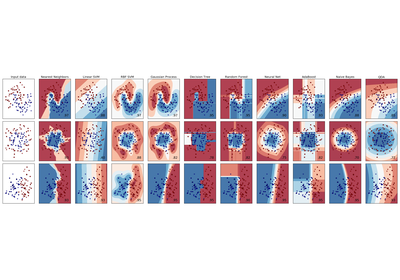Classifier comparison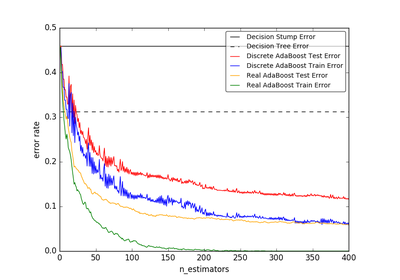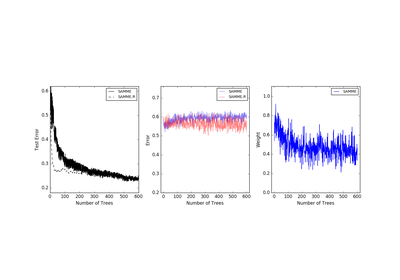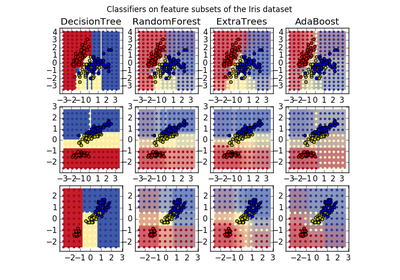Plot the decision surfaces of ensembles of trees on the iris dataset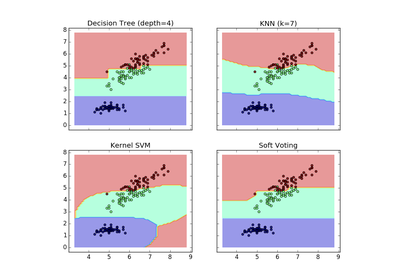Plot the decision boundaries of a VotingClassifier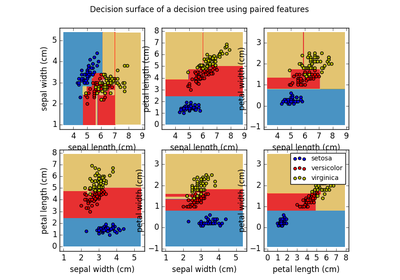Plot the decision surface of a decision tree on the iris datasetUnderstanding the decision tree structure